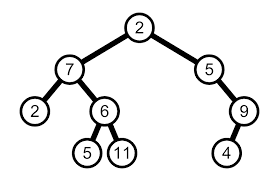# Lowest Common Ancestor (LCA) of a Binary Tree

#### Problem Statement

Given a Binary Tree with some nodes find the lowest common ancestor (LCA) of given any two Nodes of the Binary Tree.

LCA of the Binary Tree is the deepest most node which has both the given nodes as its descendants and it is closest to both of the given nodes.

To know more about LCA click here

#### ExampleFigure to depict LCA of given Two Nodes

In the Above Example

LCA (7,9) =  2

LCA (2,6) = 7

LCA (6,4) = 2

Approach

The basic idea to solve the above problem is to check if while traversing the Binary Tree we have found any one of the given node.If found any of the given node simply return it.There can arise 4 cases while traversing the trees

1. Any one value is found on the left sub tree of the current node and other value is found on right sub tree of the current node.In this case the current node is the LCA.
2. Left sub tree founds any one value but right sub tree doesn't found any value.In this case LCA will be the node which the left sub tree returns.
3. Right sub tree founds any one value but left sub tree doesn't found any value.In this case LCA will be the node which the right sub tree returns.
4. If at any node ,if its both left and right sub tree doesn't finds any one of the given value and both of them returns null then neither this current node is not LCA nor any of its left and right sub tree has LCA.So return null.

#### Implementation

Below is the implementation of the above approach in Java

class Node{

int key;
Node left, right;

public Node(int item)
{
key = item;
left = right = null;
}
}
class BinaryTree
{
Node root;

BinaryTree(int key)
{
root = new Node(key);
}

BinaryTree()
{
root = null;
}

public static void main(String[] args)
{
BinaryTree tree = new BinaryTree();

tree.root = new Node(2);
tree.root.left = new Node(7);
tree.root.right = new Node(5);
tree.root.left.left = new Node(2);
tree.root.left.right = new Node(6);
tree.root.left.right.left = new Node(5);
tree.root.left.right.right = new Node(11);
tree.root.right.right = new Node(9);
tree.root.right.right.left = new Node(4);
System.out.println(lowestCommonAncestor(tree.root,tree.root.left.left,tree.root.left).key);
}
public static Node lowestCommonAncestor(Node root, Node p, Node q) {

if(root==null)
{
return null;
}
if(root==p || root==q)
{
return root;
}

Node left=lowestCommonAncestor(root.left,p,q);
Node right=lowestCommonAncestor(root.right,p,q);

if(left==null && right==null)
{
return null;
}
if(left!=null && right!=null)
{
return root;
}
if(left!=null && right==null)
{
return left;
}
return right;
}
}

#### Output

2

For more Data structures and algorithms related problems visit icoders